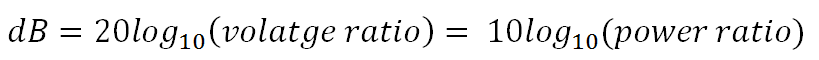#### dB → V → P ratio toolThe equation below shows the relationship between a decibel value and a linear voltage/power ratio. This calculator is can be used in one of three ways:

• A decibel value can be specified and the linear voltage and power ratios will be returned
• A linear voltage ratio can be specified and the decibel value together with the linear power ratio is returned
• A linear power ratio can be specified and the decibel value together with the linear voltage ratio is returned
•